Next: Forbidden Transitions Up: Time-Dependent Perturbation Theory Previous: Transitions in Hydrogen

# Intensity Rules

Now, we know, from Sect. 12.8, that when we take electron spin and spin-orbit coupling into account the degeneracy of the six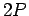states of the hydrogen atom is broken. In fact, these states are divided into two groups with slightly different energies. There are four states characterized by the overall angular momentum quantum number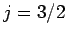--these are called the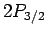states. The remaining two states are characterized by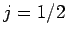, and are thus called the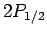states. The energy of thestates is slightly higher than that of thestates. In fact, the energy difference is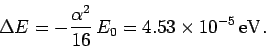(1162)

Thus, the wavelength of the spectral line associated with thetransition in hydrogen is split by a relative amount(1163)

Note that this splitting is much greater than the natural line-width estimated in Eq. (1161), so there really are two spectral lines. How does all of this affect the rate of thetransition?

Well, we have seen that the transition rate is independent of spin, and hence of the spin quantum number, and is also independent of the quantum number. It follows that the transition rate is independent of the-component of total angular momentum quantum number. However, if this is the case, then the transition rate is plainly also independent of the total angular momentum quantum number. Hence, we expect the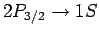and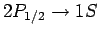transition rates to be the same. However, there are fourstates and only twostates. If these states are equally populated--which we would certainly expect to be the case in thermal equilibrium, since they have almost the same energies--and since they decay to the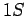state at the same rate, it stands to reason that the spectral line associated with thetransition is twice as bright as that associated with thetransition.Next: Forbidden Transitions Up: Time-Dependent Perturbation Theory Previous: Transitions in Hydrogen
Richard Fitzpatrick 2010-07-20